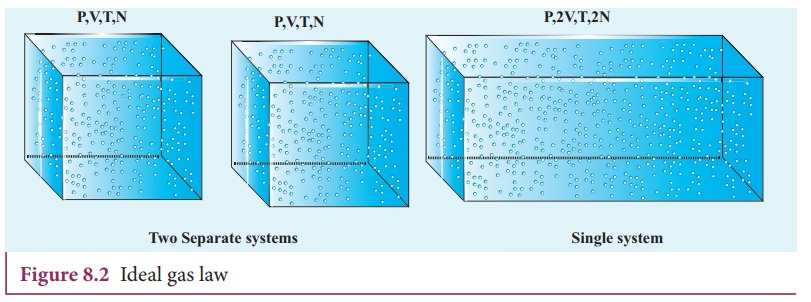Home | | Physics 11th std | Boyle‚Äôs law, Charles‚Äô law and ideal gas law - Thermal Properties of Matter

# Boyle‚Äôs law, Charles‚Äô law and ideal gas law - Thermal Properties of Matter

Thermal Properties Of Matter - Boyle‚Äôs law, Charles‚Äô law and ideal gas law

THERMAL PROPERTIES OF MATTER

Boyle‚Äôs law, Charles‚Äô law and ideal gas law

For a given gas at low pressure (density) kept in a container of volume V, experiments revealed the following information.

¬∑           When the gas is kept at constant temperature, the pressure of the gas is inversely proportional to the volume. P ‚àù 1/V. It was discovered by Robert Boyle (1627-1691) and is known as Boyle‚Äôs law.

¬∑           When the gas is kept at constant pressure, the volume of the gas is directly proportional to absolute temperature. V ‚àùT . It was discovered by Jacques Charles (1743-1823) and is known as Charles‚Äô law.

¬∑           By combining these two equations we have

PV = CT. Here C is a positive constant.

We can infer that C is proportional to the number of particles in the gas container by considering the following argument. If we take two containers of same type of gas with same volume V, same pressure P and same temperature T, then the gas in each container obeys the above equation. PV = CT. If the two containers of gas is considered as a single system, then the pressure and temperature of this combined system will be same but volume will be twice and number of particles will also be double as shown in Figure 8.2For this combined system, V becomes 2V, so C should also double to match with the ideal gas equation P(2V) / T = 2C . It implies that C must depend on the number of particles in the gas and also should have the dimension of [PV/T] = JK-1. So we can write the constant C as k times the number of particles N.

Here k is the Boltzmann constant (1.381√ó10‚àí23 JK‚àí1) and it is found to be a universal constant.

So the ideal gas law can be stated as followsThe equation (8.1) can also be expressed in terms of mole.

Suppose if a gas contains Œº mole of particles then the total number of particles can be written aswhere NA is Avogadro number (6.023 √ó1023mol-1)

Substituting for N from equation (8.2), the equation (8.1) becomes

PV = Œº NAkT. Here NAk=R called universal gas constant and its value is 8.314 J /mol. K.

So the ideal gas law can be written for Œº mole of gas asThis is called the equation of state for an ideal gas. It relates the pressure, volume and temperature of thermodynamic system at equilibrium.

### EXAMPLE 8.2

A student comes to school by a bicycle whose tire is filled with air at a pressure 240 kPa at 27¬∞C. She travels 8 km to reach the school and the temperature of the bicycle tire increases to 39¬∞C. What is the change in pressure in the tire when the student reaches school?### Solution

We can take air molecules in the tire as an ideal gas. The number of molecules and the volume of tire remain constant. So the air molecules at 27¬∞C satisfies the ideal gas equation P1V1 = NkT1 and at 39¬∞C it satisfies P2V2 = NkT2

But we know### EXAMPLE 8.3

When a person breaths, his lungs can hold up to 5.5 Litre of air at body temperature 37¬∞C and atmospheric pressure (1 atm =101 kPa). This Air contains 21% oxygen. Calculate the number of oxygen molecules in the lungs.### Solution

We can treat the air inside the lungs as an ideal gas. To find the number of molecules, we can use the ideal gas law.

PV = NkT

Here volume is given in the Litre. 1 Litre is volume occupied by a cube of side 10 cm. 1Litre = 10cm √ó 10cm √ó 10cm = 10-3 m3Only 21% of N are oxygen. The total number of oxygen moleculesNumber of oxygen molecules = 2.7 √ó 1022 molecules

### EXAMPLE 8.4

Calculate the volume of one mole of any gas at STP and at room temperature (300K) with the same pressure 1 atm.

### Solution:

Here STP means standard temperature (T=273K or 0¬∞C) and Pressure (P=1 atm or 101.3 kPa)

We can use ideal gas equation V = ŒºRT / P.

By substituting the values=22.4 √ó 10-3 m3

We know that 1 Litre (L) = =10-3m3. So we can conclude that 1 mole of any ideal gas has volume 22.4 L.

By multiplying 22.4L by 300/273KK we get the volume of one mole of gas at room temperature. It is 24.6 L.

### EXAMPLE 8.5

Estimate the mass of air in your class room at NTP. Here NTP implies normal temperature (room temperature) and 1 atmospheric pressure.### Solution

The average size of a class is 6m length, 5 m breadth and 4 m height. The volume of the room V = 6 √ó 5 √ó 4 = 120m3. We can determine the number of mole. At room temperature 300K, the volume of a gas occupied by any gas is equal to 24.6L.

The number of mole Œº=‚âà4878 mol .

Air is the mixture of about 20% oxygen, 79% nitrogen and remaining one percent are argon, hydrogen, helium, and xenon. The molar mass of air is 29 gmol-1.

So the total mass of air in the room m = 4878 √ó 29 = 141.4kg.

Study Material, Lecturing Notes, Assignment, Reference, Wiki description explanation, brief detail
11th Physics : UNIT 8 : Heat and Thermodynamics : Boyle‚Äôs law, Charles‚Äô law and ideal gas law - Thermal Properties of Matter |